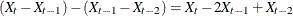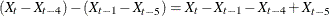### Stationarity and Differencing

The state space model used by the STATESPACE procedure assumes that the time series are stationary. Hence, the data should be checked for stationarity. One way to check for stationarity is to plot the series. A graph of series over time can show a time trend or variability changes.

You can also check stationarity by using the sample autocorrelation functions displayed by the ARIMA procedure. The autocorrelation functions of nonstationary series tend to decay slowly. See Chapter 7: The ARIMA Procedure, for more information.

Another alternative is to use the STATIONARITY= option in the IDENTIFY statement in PROC ARIMA to apply Dickey-Fuller tests for unit roots in the time series. See Chapter 7: The ARIMA Procedure, for more information about Dickey-Fuller unit root tests.

The most popular way to transform a nonstationary series to stationarity is by differencing. Differencing of the time series is specified in the VAR statement. For example, to take a simple first difference of the series X, use this statement:

```   var x(1);
```

In this example, the change in X from one period to the next is analyzed. When the series has a seasonal pattern, differencing at a period equal to the length of the seasonal cycle can be desirable. For example, suppose the variable X is measured quarterly and shows a seasonal cycle over the year. You can use the following statement to analyze the series of changes from the same quarter in the previous year:

```   var x(4);
```

To difference twice, add another differencing period to the list. For example, the following statement analyzes the series of second differences:

```   var x(1,1);
```

The following statement analyzes the seasonal second difference series:

```   var x(1,4);
```

The series that is being modeled is the 1-period difference of the 4-period difference:.

Another way to obtain stationary series is to use a regression on time to detrend the data. If the time series has a deterministic linear trend, regressing the series on time produces residuals that should be stationary. The following statements write residuals of X and Y to the variable RX and RY in the output data set DETREND.

```   data a;
set a;
t=_n_;
run;

proc reg data=a;
model x y = t;
output out=detrend r=rx ry;
run;
```

You then use PROC STATESPACE to forecast the detrended series RX and RY. A disadvantage of this method is that you need to add the trend back to the forecast series in an additional step. A more serious disadvantage of the detrending method is that it assumes a deterministic trend. In practice, most time series appear to have a stochastic rather than a deterministic trend. Differencing is a more flexible and often more appropriate method.

There are several other methods to handle nonstationary time series. For more information and examples, see Brockwell and Davis (1991).